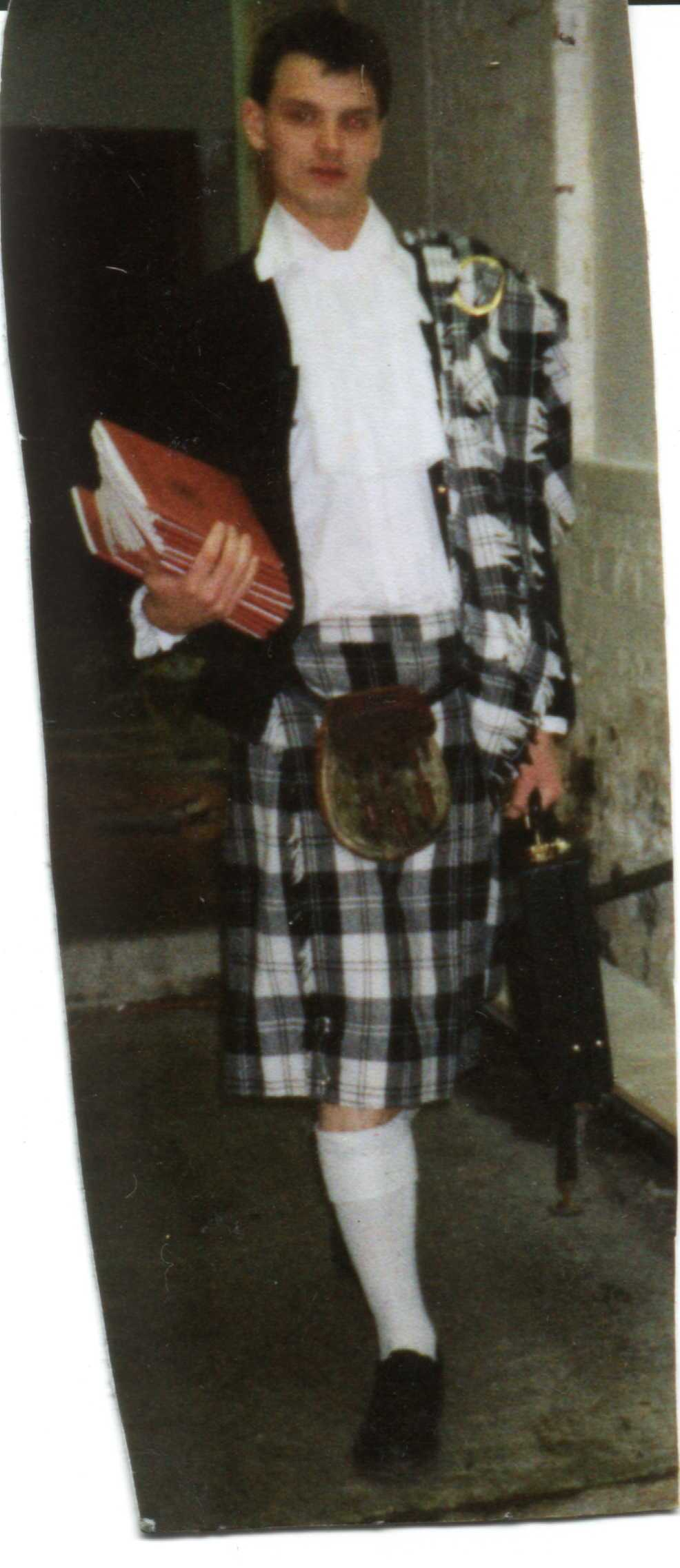EVIL

Especially the scottish ones

The universe works on mathematics, and mathematics works on the Universe, ie the only reason Maths works is because the universe operates on mathematic principles. Therefore, if it was the work of some being, that being must have been a mathematical being. This means that God must be a mathematician, and it is reasonable to assume that his angels were also educated in the principles of maths. Lucifer, being the most trusted of the angels, would have have been better educated and more knowledgeable than the others. Following his fall from grace, he became the source of all Evil in the universe- in other words, the works of Satan are the works of a Mathematician. Maths teachers then must be Evil in some way as Evil is a force of Satan.
So why are scottish maths teachers more evil than the rest? Well, scottish maths teachers tend to be even better qualified than regular maths teachers, so their mathematical, and hence satanic , powers are greater!